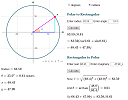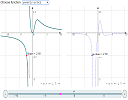Search IntMath
Close

450+ Math Lessons written by Math Professors and Teachers

5 Million+ Students Helped Each Year

1200+ Articles Written by Math Educators and Enthusiasts

Simplifying and Teaching Math for Over 23 Years

# IntMath Newsletter: Applets, Digital Einstein

By Murray Bourne, 15 Jan 2015

15 Jan 2015

1. New interactive applets
2. Resource: Digital Einstein
3. Math puzzles
4. Final thought - Passionately curious

I received a delightful mail from Den shortly after the last Newsletter went out. This is what he said:

Again Mr. Bourne let me compliment you on producing such a high quality newsletter. Yours is the only news letter that I subscribe to.

Thank you very very much for producing such valuable content for math tutors!

Sincerely,
Den Ducoff

## 1. New interactive applets

### (a) Polar to rectangular calculatorHere's a new complex numbers online calculator that converts polar form to rectangular form and vice-cersa. It also displays a graph so the concepts make more sense. See Polar to Rectangular Online Calculator

### (b) Differentiation graphsThis applet allows you to explore the graph of a derivative involving discontinuities. You can animate the path of a point on both the original graph and the derivative. Differentiation interactive applet

This next applet is related, but involves continuous functions only:

## 2. Digital EinsteinPrinceton University has released The Collected Papers of Albert Einstein, which give a fascinating insight into the original thinking of this oft-quoted genius.

It's not all about the Theory of Relativity, of course. The following quote caught my eye, because he's talking about a subject that trips up most beginner calculus students — what happens to those "infinitely small quantities" as they "tend to zero", and which are vital to how calculus works? Eseentially, he says not to worry too much about it:

Whether one, along with Leibnitz, Poisson, Herbert, et al., seriously wants to take the infinitesimally small for a truly indivisible element, or one wants, along with others, to take it only for a useful fiction, so as thereby supposedly to eliminate all metaphysical difficulties, and conveniently and quickly introduce the calculus, is irrelevant for the calculus, for the one as much as the other leads to the goal.

[From Volume 1: The Early Years, 1879-1902 (English translation supplement) Page 4]

## 3. Math puzzles

The puzzle in the last IntMath Newsletter asked about the cost of a chocolate bar. The correct answer with explanation was given by Chris, Thomas, Tomas, and Francisco.

New math puzzle: The sequence xn is defined as:

xn = n3 − 9n2 + 631 (where n is an integer)

What is the highest value of n such that xn > xn+1?

Correction: Last mail (10 Dec 2014 edition), I missed giving proper recognition to all those who responded to the exponential and log functions puzzle (in the 28 Nov 2014 Newsletter). I corrected it here.

## 4. Final thought - Passionately curious

On the home page of Digital Einstein (mentioned above), they've included a great Einstein quote:

"I have no special talents. I am only passionately curious." [Einstein]

A truly intelligent person is one who is keen to learn as much as they can about a wide variety of topics, and who asks a lot of questions. I've also observed that the best teachers provide good role models for their students' learning. They question things, are willing to admit they don't know something, and have good ideas on how they might find the answers.

Until next time, enjoy whatever you learn.

1. Tomas Garza says:

The function f(x) = x^3 - 9x^2 + 631, for real x, is increasing when its derivative is positive, i.e., 3x^2 - 18x > 0, and this is true when x^2 > 6x, or x > 6. For integer values of x, f(n) > f(n+1) holds for all n > 6. So it´s a matter of inspecting one by one the first five terms and conclude that, since f'(5) = 531 and f(6) = 523, n = 5 is the solution to the problem.

2. Helena Otto says:

xn = n3 – 9n2 + 631
xn + 1 = (n + 1)3 – 9(n + 1)2 + 631
= n3 + 3n2 + 3n + 1 – 9n2 – 18n – 9 + 631
= (n3 – 9n2 + 631) + 3n2 – 15n – 9
= xn + 3n2 – 15n – 9
xn – xn + 1 = - 3n2 + 15n + 9 (xn > xn + 1)
For the RHS to be a maximum n = -b/2a = -15/2(-3) = 2.5

3. Thomas A Buckley says:

Happy numbers for all in the New Year.
The highest value of n to satisfy the problem is 5.

Solution:
Equating the 1st derivative to 0, and solving for n yields n=0 or+6
These are the turning points. A + value indicates an upward concave,
while n= 0 or a -value indicates a downward concave.
The 2nd derivative yields n = +3 as the point of inflection having a -slope,
and as expected lies between the turning points n=0 and n=6 found above.

Try to visualise this area of the equation. Its similar to the + half of a
sine wave followed by the - half.

OK so far? But where is the answer?
n=6 is the final value in an incrementing range of n values
where x is diminishing. 6 being an integer is convenient.
So 6 - 1= 5 is the previous integer = the ANSWER

Proof :
In Xn = n^3 - 9n^2 + 631, solving for
When n = 5, X =531. ie Xn =X5
and when n = 6, X=523. ie Xn+1=X6. Note 531 > 523.

The value of Xn > Xn+1,
over the highest integer values of n between 5 and 6.

4. Dineth says:

it's 5.
can be obtained by solving the equation by assuming Xn and Xn+1 are equal.
(n^3)-9*(n^2)+631=(n+1)^3-9(n+1)^2+631
n=5.48

it can be visualized easily by a graph.
n X
1 623
2 603
3 577
4 551
5 531
6 523
7 533
8 567

(this happens because - and + terms, after that this will never happen as gap only increase. so this is the highest value.)

5. Nicos Mavrommatis says:

Let Xn=n^3 -9n^2 + 631 (1), then X(n+1)=(n+1)^3 -9(n+1)^2 + 631 (2).
Let Dn=X(n+1)-Xn < 0 (3). Using (1) & (2) in (3):
Dn=(n+1)^3 -9(n+1)^2 +631 -n^3 +9n^2 -631
=> Dn= 3n^2 -15n -8 <0 (4)
The roots of Dn are: n1=-0.486 & n2=5.486
The inequation (4) is true, when -0.486<n<5.486
The maximum integer in this specific interval is 5.
So, n(max)=5

6. hamid zandi says:

xn=3*n^3-9*n^2+631;xn+1=(n+1)^3-9*(n+1)^2+631
xn>xn+1=> 3*n^2-15*n-8~-0.4<n highest content of n is = 5

7. Michael El-Jiz says:

x(n) > x(n+1)

by expansion, you end up with

-3n^2 + 15n +8 > 0
to which the roots are
n ~= -0.4 and 5.48

since a < 0, then the parabola is positive in between the roots.

The largest integer value of n which satisfies the condition is n = 5.

8. Henry says:

n=5 either by trial & error or differentiate the function to find the turning point at n=6 leaving n=5 as the solution.

9. Francis Kisner says:

The sequence xn is defined as:
xn = n^3 − 9n^2 + 631 (where n is an integer)
What is the highest value of n such that xn > xn+1?

Although this is defined here as an integer sequence, the definition could be viewed as a function. The first derivative of the function set to 0 is 0 = 3n^2 - 18n from which n = 0 or n = 6. These are the local max and local min points of the function. At n = 6, the value of x6 is less than the value at x7. At n = 5, the value of x5 is greater than the value of x6. From 6 onward, the function continues to increase so xn will always be less than xn+1. That leave n=5 as the answer.

10. Valentine says:

8.The function is increasing after 8.

11. Helena Nayar says:

Answer is 5. Since it is a decreasing sequence, the difference between the nth and the (n+1)th term is negative. This difference is a quadratic in n. Solving the 'less-than' inequality in n, we get the value.

12. Francisco Gudiño says:

Dear Mr Bourne.
In the sequence n^3-9*n^2+631.
d(n^3-9*n^2+631)/dn=3*n^2-18*n.
3*n(n-6)=0. Then: n=0 or n=6.
Similarly,d(3*n^2-18*n)=6*n-18.
For n=0;6*n-18=-18. Then P(0;631) is a maximum point.
For n=6;6*n-18=18. Then P(6;523) is a minimum point.
Thus the highest value of n such that Xn>X(n+1) is 5.
I don't speak English.I hope you understand me.
Best regards.

13. PAVITHRA P says:

The sequence〖 x〗_n= n^3-9n^2+631
Let us take n=0,
x_0= 0^3-9(0)^2+631
x_0= 631

Let us take n=1,
x_1= 1^3-9(1)^2+631
x_1= 622
Let us take n=-1,
x_(-1)= 〖-1〗^3-9(-1)^2+361
x_(-1)= 621

therefore we can conclude,
the maximum value of the sequence 〖 x〗_n= n^3-9n^2+631 is obtained by only n=0
and the previous explanations, x_n > x_(n+1) is proved

14. Dineth says:

So, we are excited to see the next newsletter. Hope you are cooking good one this time too.

### Comment Preview

HTML: You can use simple tags like <b>, <a href="...">, etc.

To enter math, you can can either:

1. Use simple calculator-like input in the following format (surround your math in backticks, or qq on tablet or phone):
a^2 = sqrt(b^2 + c^2)
(See more on ASCIIMath syntax); or
2. Use simple LaTeX in the following format. Surround your math with $$ and $$.
$$\int g dx = \sqrt{\frac{a}{b}}$$
(This is standard simple LaTeX.)

NOTE: You can mix both types of math entry in your comment.